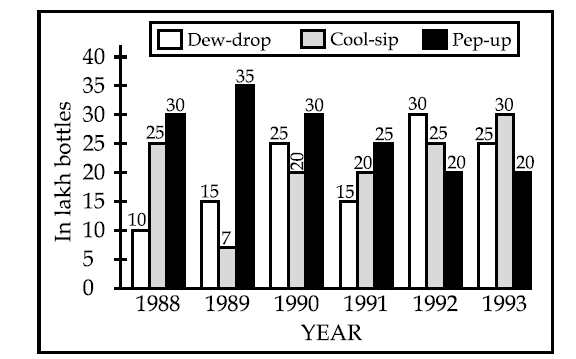UGC-NET/SET-PAPER-I(2004-DEC) - MODEL PAPER-34
ONLINE TEST NET/SET PAPER-1
Email address *
Study the following graph and answer the questions from 31 to 33:
2 points32. In the case of which soft drink was the average annual sale maximum during the period 1988 - 1993.
2 points
33. What was the approximate percent drop in the sale of Pep - up in 1990 over its sale in 1989 ?
2 points
34. The “Report on Currency and Finance” for each of the financial year in India is published by :
2 points
35. The number of students in two classes A and B and the respective “mean” of the marks obtained by each of the class are given in the following table :
2 points
36. ICT stands for :
2 points
37. Which of the following statements is NOT correct ?
2 points
38. Which of the following is the appropriate definition of Information Technology?
2 points
39. Which of the following statements is correct?
2 points
40. Which of the following is correct statement?
2 points